Interface and Application Programming

Processing

Processing

Color Sensor Circuit

My setup and pinout is shown below:
Color Sensor --> Arduino
RED --> Pin 9
GREEN --> Pin 10
BLUE --> Pin 11
WHITE --> Pin 6
ANALOG --> Pin A0
VCC --> VCC
GND --> GND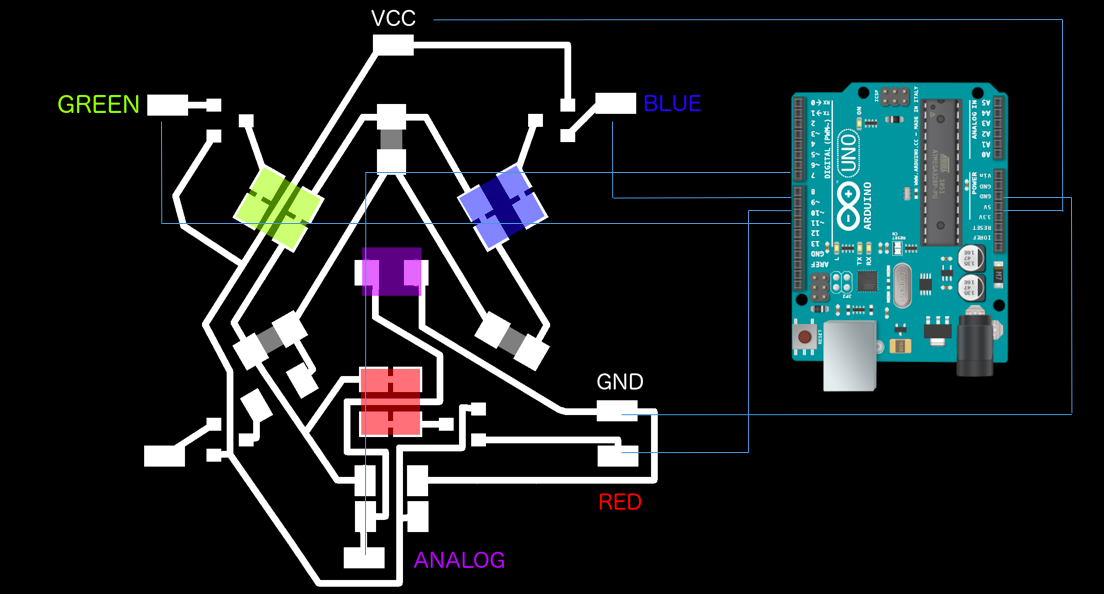Connect the Arduino Uno to the USB port. Open the Arduino software select the port connected to the arduino (Tools -> Port). Load the following code into a sketch and upload the sketch.

```int LED = {9,10,11,6},i, j ; // DECLARE R G B PINS void setup() { Serial.begin(9600); for(i=0;i<4;i++) // set LED pins to OUTPUT pinMode(LED[i], OUTPUT); } void loop() { for(j = 0 ; j < 4 ; j++) // CYCLE PINS and set pins low. { analogWrite(LED[j],0); } for(j = 0 ; j < 3 ; j++) // CYCLE PINS { for(i =0 ; i < 255 ; i++) // CYCLE PWM VALUES - this changes the brightness of the LED from 0 - off to 255 - completely on. { analogWrite(LED[j],i); Serial.println(1024-analogRead(0)); // PRINT VALUES - to view these open the serial monitor. delay(10); } analogWrite(LED[j],0); } }```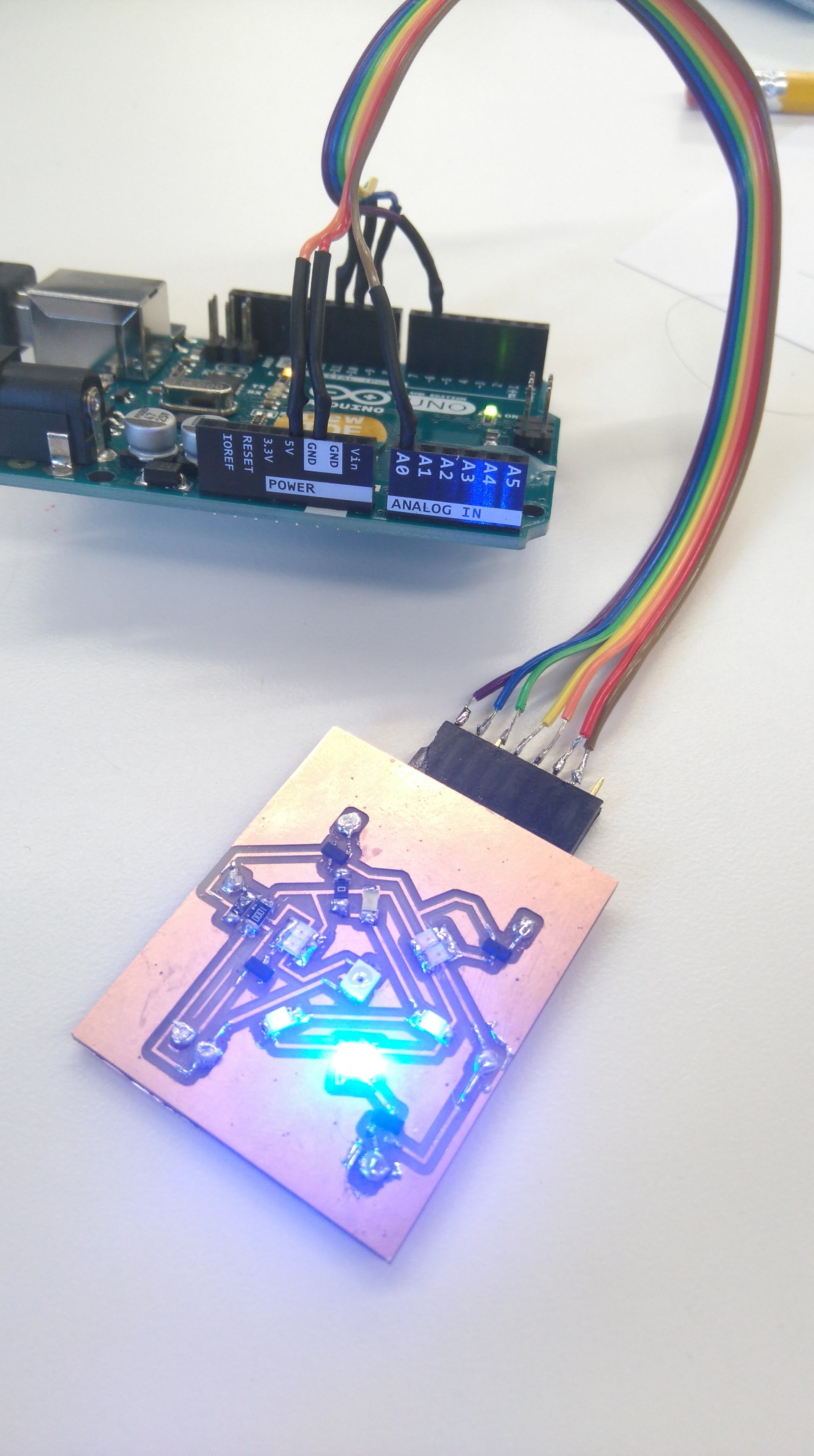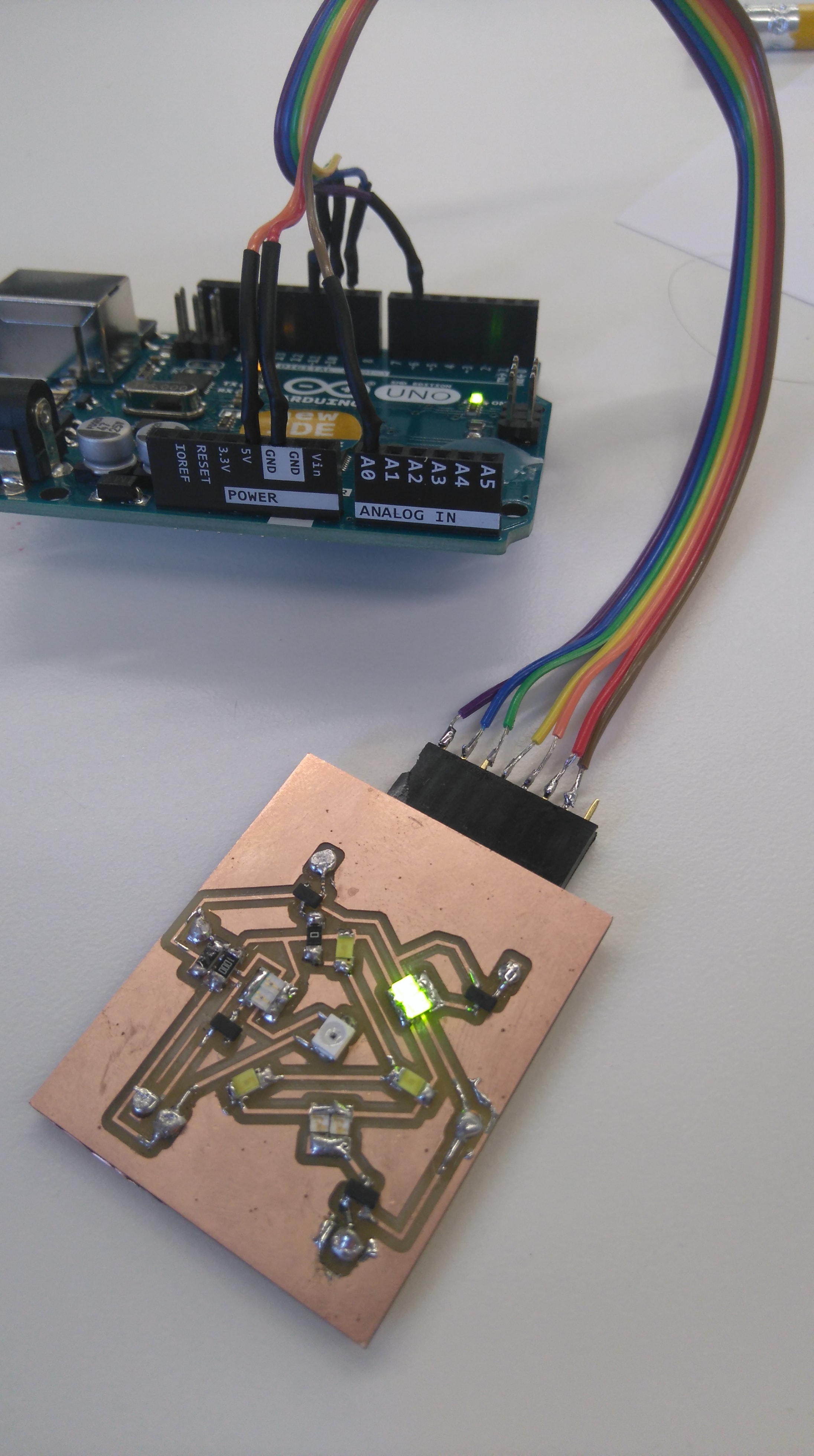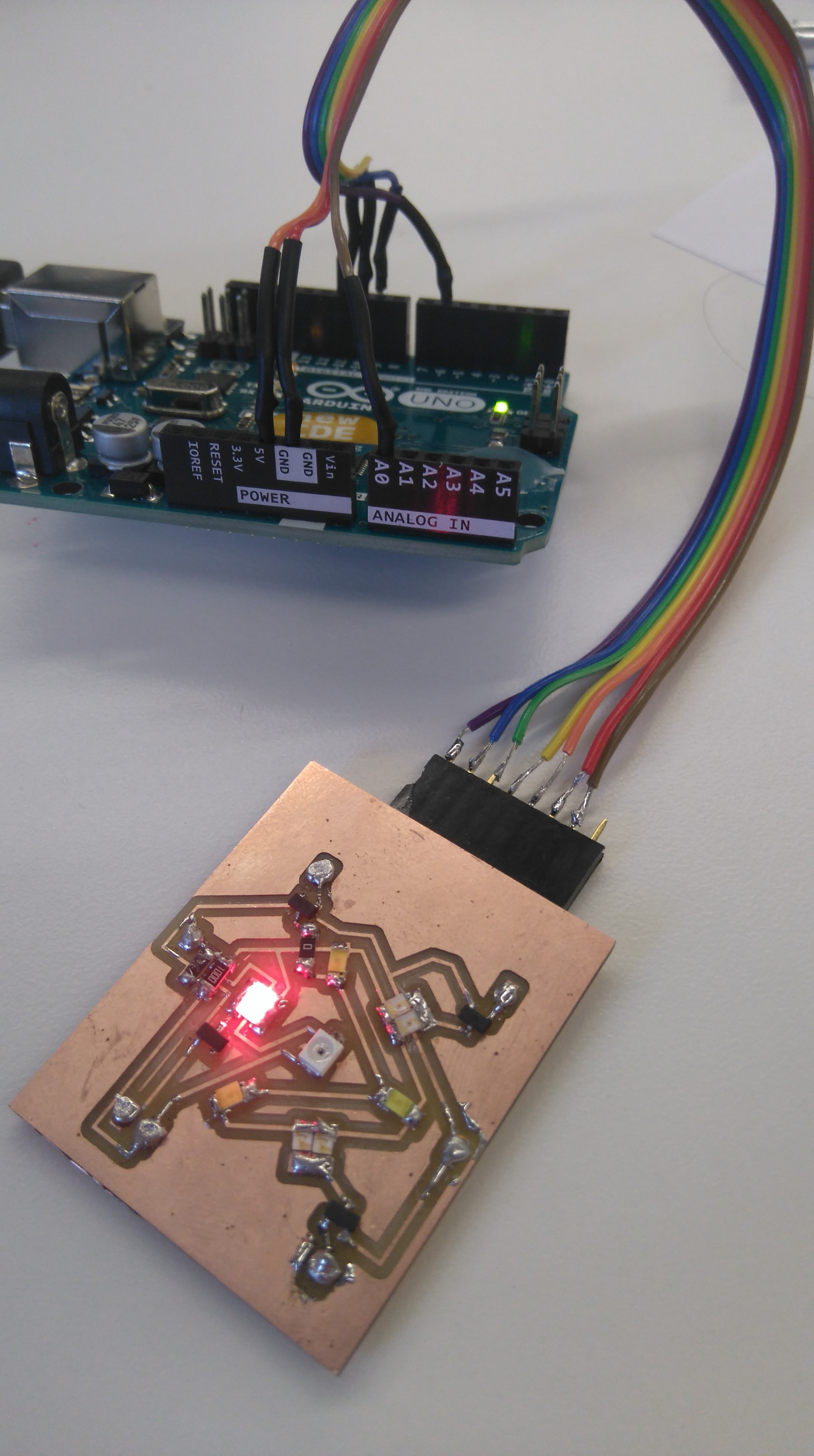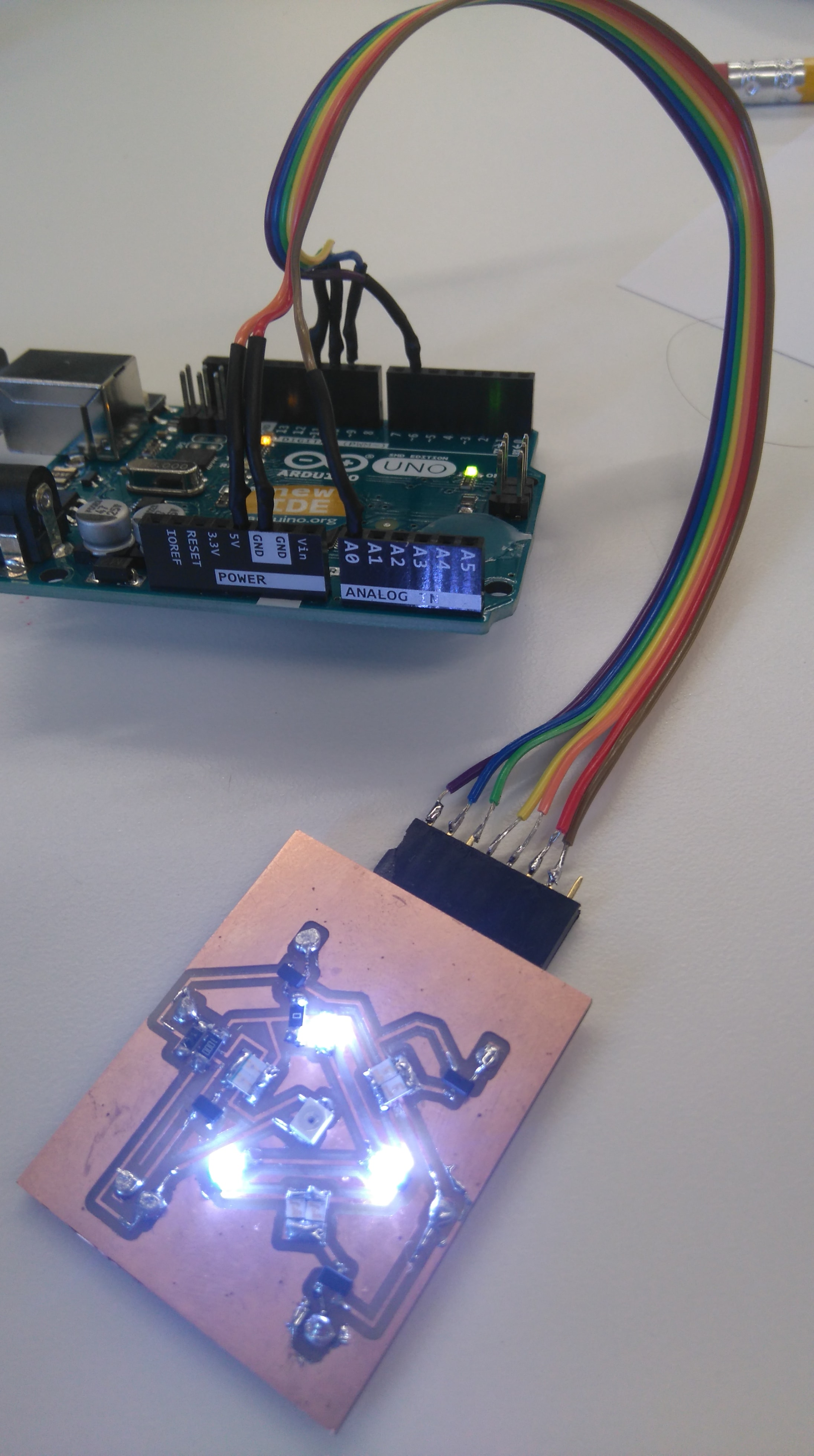You should see the lights alternating and each color increasing in brightness.

I decided to use processing to read from the analog 0 pin of the arduino. I downlowded processing and began to get to work trying to understand how it works. I ran into a couple of problems that took quite a while to figure out:
1) Firstly my Phototransistor was soldered on backwards and I was therefor not getting any readings that made sense.
2) You can only draw lines in the draw function.

Here is my processing code ```import processing.serial.*; Serial myPort; float xPos = 20,prevtime=0; float inByte; String inString; int i = 0; float inBytetotal = 0; float inByteave = 0; int ave = 0; void setup () { size(700, 700); //Set canvas size. // List all the available serial ports printArray(Serial.list()); myPort = new Serial(this, Serial.list(), 9600); myPort.bufferUntil('\n'); background(255); //Set background colour of canvas } void draw () { // draw the line: stroke(255, 0, 0); line(xPos, height, xPos, height - inByteave); inBytetotal = 0; inByteave = 0; if (xPos >= width) { xPos = 0; background(255); } else { xPos++; } } void serialEvent (Serial myPort) { inString = myPort.readStringUntil('\n'); if (inString != null) { inString = trim(inString); inByte = float(inString); inByte = map(inByte, 0.0, 1024.0, 0.0, 700.0); //print(inByte); //print('\n'); //print(xPos); //print('\n'); if(i { inBytetotal=inBytetotal+inByte; i++; } if(i==ave) { inBytetotal = inBytetotal+inByte; inByteave=(inBytetotal/(1+1)); i=0; } } }```

The video below highlights a couple of things:
1) The Green light is extremely dim and will need to be replaced by a more effective Green LED.
2) The oscillations that can be seen I believe are a result of the PWM frequency and the frequency that readings are measured at - I might have to put a capacitor across the Phototransistor pins...
3) I will need to calibrate light sensor.